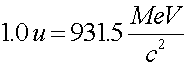Masses and energy

According to the relation E = mc2, when the energy of a system changes, the mass changes as well. This is true for atomic, chemical or nuclear processes. Although many associate E=mc2 with nuclear process, mass is converted to energy in chemical reactions as well. Although in nuclear processes, the percentage of mass change is much more detectable than in chemical processes, it is still only a fraction of a percent.

The mass of nuclear states is often measured in mass units. One atomic mass unit (noted by a.u. or amu or u) corresponds to one twelth the mass of a carbon-12 nucleus.When nuclei decay, they always go to a lower energy state. The excess energy not contained in nuclei or nucleons, the Q-value, is taken up by photons, neutrinos or electrons. By summing the before and after masses of a reaction, one can calculate the Q-value.

To calculate the Q-value in MeV given the mass change, Dm, you simply multiply the mass change (in u) by 931.5 to get the energy released in the reaction in MeV.

Nuclear physics index      examples        Lecture index